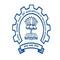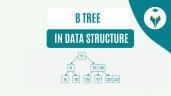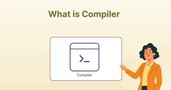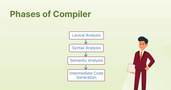Know the Difference Between Algorithm and Program

# Know the Difference Between Algorithm and Program

clickHere
Updated on Aug 9, 2023 12:46 IST

An algorithm can be defined as a systematic approach to solving a specific problem. An understanding of algorithms is essential for programmers to program more efficiently. It is equally important for programmers to understand the difference between algorithms and programs for optimizing a program according to the available resources. In this article, we will look at each term’s definitions and explore the difference between algorithm and program.

Explore the Best Programming Courses

## What is an Algorithm?

An algorithm is a step-by-step and logical approach that defines a systematic process for computers to solve a specific problem. It consists of a set of rules defining how a task will be executed to get the expected results. Algorithms are conceptual and can be described using language or flowcharts. We can implement them in different programming languages​​. When we use a computer to solve a specific problem, we need to tell these steps to the solution clearly to the computer.

For example, here’s an algorithm to add two numbers:

#### Characteristics of an Algorithm

1. An algorithm should be unambiguous.
2. It should take well-defined inputs.
3. The algorithm should take well-defined outputs.
4. It should be simple, generic, and can be executed using the available resources.
5. The algorithm must be finite.
6. It must be language-independent.

Also Read: Understanding Data Structures and Algorithms in Java

#### Types of Algorithms

Here are the most popular type of algorithms:

1. Recursive Algorithm – The Algorithm will call itself repeatedly until the problem is solved. Example: Computing factorials
2. Greedy Algorithm – Algorithms that are used for solving optimization problems. Example: Huffman tree
3. Brute Force Algorithm – It is one of the simplest algorithms in the concept. It iterates all possible solutions to search for one or more possible solutions that may solve a function. Example: Matching algorithm
4. Backtracking Algorithm – This algorithm follows an incremental approach to finding a solution to a problem. The algorithm will solve problems recursively. It will find a solution to a problem by solving one piece of the problem at a time. If one of the solutions fails, it is removed, and the algorithm backtracks to find another solution. Example: Queens Problem
5. Divide and Conquer Algorithm – As the name suggests, the algorithm is divided into two parts. The first part divides the problem into smaller subproblems of the same type. In the second part, these smaller problems are solved and then combined to produce the final solution to the problem. Example: Merge sort

## What is a Program?

A program is a set of instructions a computer follows to perform a specified task. Many programming languages can be used to write computer programs. Some popular programming languages include Python, Java, C++, JavaScript, PHP, and Ruby. These high-level programming languages are human-readable and writable. These languages are converted into low-level machine languages by compilers, interpreters, and assemblers within the computer system.

A program tells the computer how to accept input, manipulate that input, and display the output in some form that humans find useful.

For Example, in a word processor:

• The input will be the characters you type from the keyboard
• The program will format the text and correct the spelling
• Well-organized will be displayed on the screen as output

Must Read: Best Resources To Learn Programming Online

## Algorithm vs Program: Difference Between Programand Algorithm

Computer algorithms solve the problem while computer programs implement them in a form that a computer can execute. Here are the main differences between algorithms and programs:

Explore Popular Data Structures and Algorithms Courses

## Conclusion

So, this was all about the difference between algorithms and programming. We learned that the main difference is between the two is that an algorithm is a step-by-step procedure for solving the problem, while programming is a set of instructions for a computer to follow to perform a task. A program could also be an implementation of code to instruct a computer on how to execute an algorithm.

## FAQs

What is the main difference between an algorithm and a program?

In todayu2019s digitalized world, all the technological advancements rely on software, which is a collection of programs. Every program is built upon a series of instructions known as an algorithm. The main difference between the two is that while computer algorithms solve the problem, computer programs implement them in a form that a computer can execute.

What is a programming algorithm?

A programming algorithm defined the steps required for the computer to solve a particular problem. The computer follows the steps and shows you the results, which are called outputs. A programming algorithm is written in simple English. It has a start, a middle part, and stop part.

What are the characteristics of a good algorithm?

The characteristics of a good algorithm are Precision - each step of the algorithm must be defined clearly; Finiteness - it will stop after executing a finite number of instructions; Input - the algorithm must take an input; Output - the algorithm must produce an output.u00a0

clickHere

Rashmi is a postgraduate in Biotechnology with a flair for research-oriented work and has an experience of over 13 years in content creation and social media handling. She has a diversified writing portfolio and aim... Read Full Bio

## Trending Technology CoursesHTML, CSS, and Javascript for Web Developers
Coursera4.4Python Training
IIT Bombay4.2Programming in Java by NPTEL
NPTEL4.6Python Fundamentals for Beginners
Great Learning4.5Starts 11 JanData/Web scraping with Python
Great Learning4.0Starts 11 Jan## Top Picks & New Arrivals